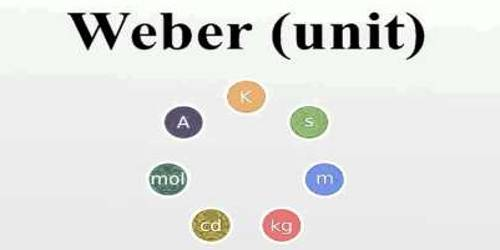# One Weber

One weber: Number of flux that charges in a coil per second for which 1-volt potential difference generates in that coil is called 1 Weber. In physics, the Weber is the SI unit of magnetic flux.

One Weber is “the magnetic flux which, linking a circuit of one turn, would produce in it an electromotive force of 1 volt if it were reduced to zero at a uniform rate in 1 second.”

The Weber was introduced as a practical electromagnetic unit = 10⁸ maxwells at the International Electrotechnical Commission in Paris in 1933, but it was not much used until the meter-kilogram-second system was generally adopted.

The Weber is named for Wilhelm E. Weber (1804-1891).

The weber is commonly expressed in a multitude of other units:

Wb = kg.m2 / s2.A = V.s = H.A = T.m2 = J/A = 108 Mx.

where Wb = weber,

V = volt, T = tesla, J = joule, m = metre, s = second, A = ampere, H = henry, and Mx = maxwell.